Скачать презентацию Chapter 14 Income Tax Considerations After-Tax Cash

b6b46c1f7ac464c3fca9959c517a014b.ppt

• Количество слайдов: 38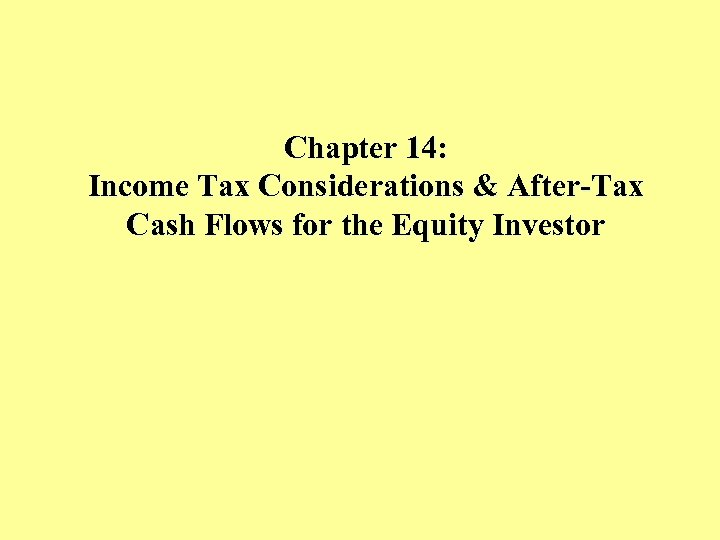Chapter 14: Income Tax Considerations & After-Tax Cash Flows for the Equity Investor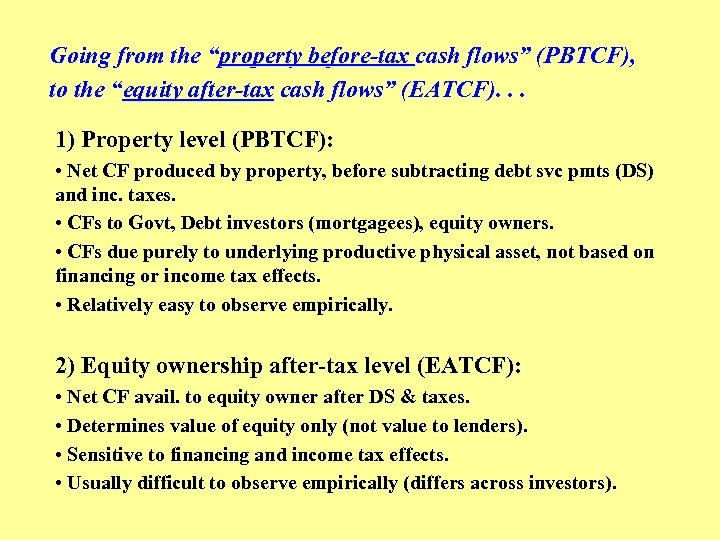Going from the “property before-tax cash flows” (PBTCF), to the “equity after-tax cash flows” (EATCF). . . 1) Property level (PBTCF): • Net CF produced by property, before subtracting debt svc pmts (DS) and inc. taxes. • CFs to Govt, Debt investors (mortgagees), equity owners. • CFs due purely to underlying productive physical asset, not based on financing or income tax effects. • Relatively easy to observe empirically. 2) Equity ownership after-tax level (EATCF): • Net CF avail. to equity owner after DS & taxes. • Determines value of equity only (not value to lenders). • Sensitive to financing and income tax effects. • Usually difficult to observe empirically (differs across investors).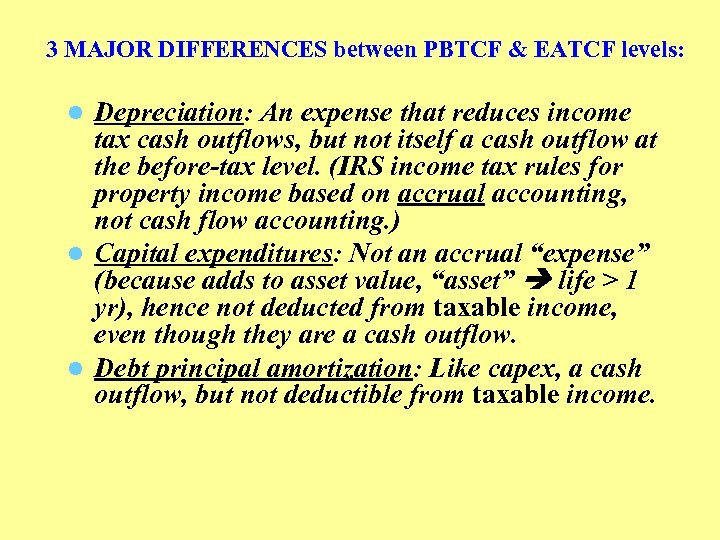3 MAJOR DIFFERENCES between PBTCF & EATCF levels: Depreciation: An expense that reduces income tax cash outflows, but not itself a cash outflow at the before-tax level. (IRS income tax rules for property income based on accrual accounting, not cash flow accounting. ) l Capital expenditures: Not an accrual “expense” (because adds to asset value, “asset” life > 1 yr), hence not deducted from taxable income, even though they are a cash outflow. l Debt principal amortization: Like capex, a cash outflow, but not deductible from taxable income. l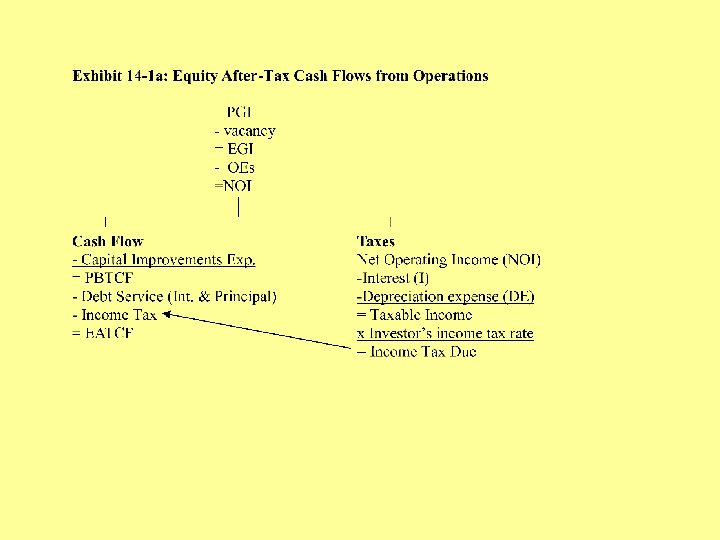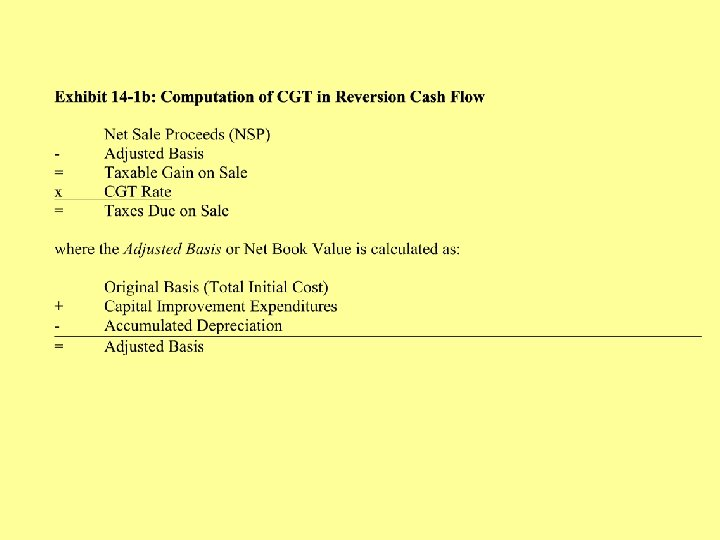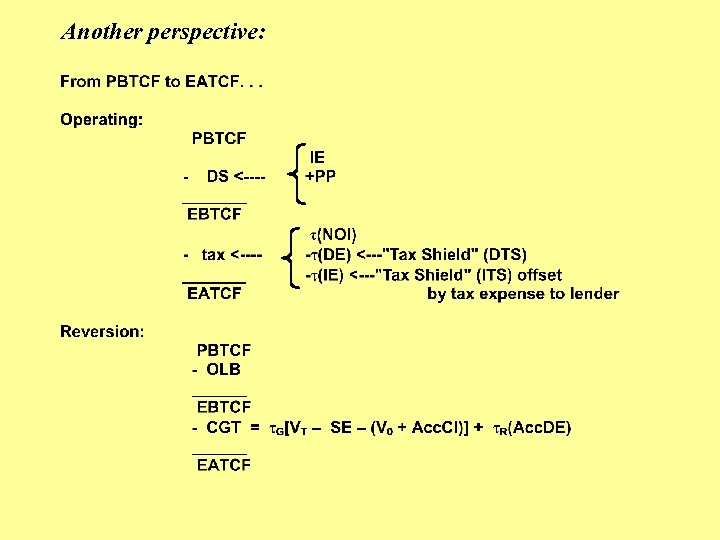Another perspective: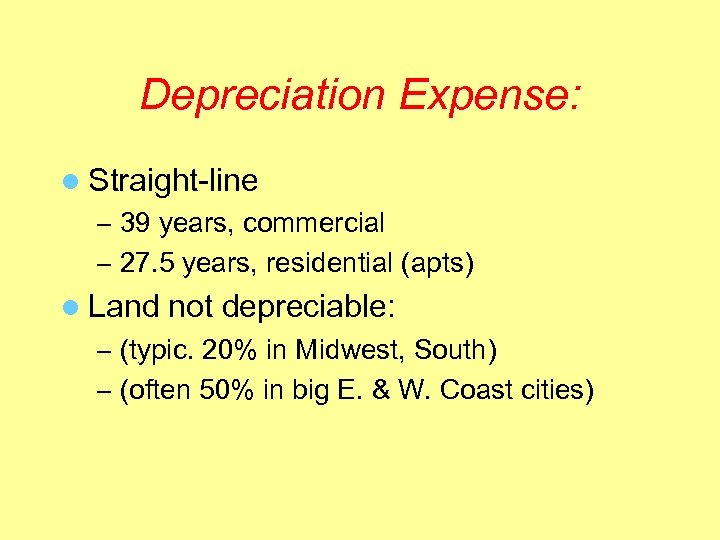Depreciation Expense: l Straight-line – 39 years, commercial – 27. 5 years, residential (apts) l Land not depreciable: – (typic. 20% in Midwest, South) – (often 50% in big E. & W. Coast cities)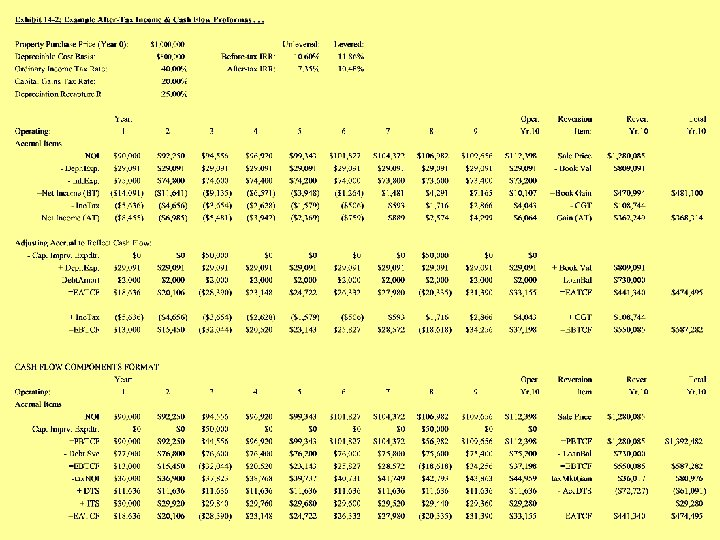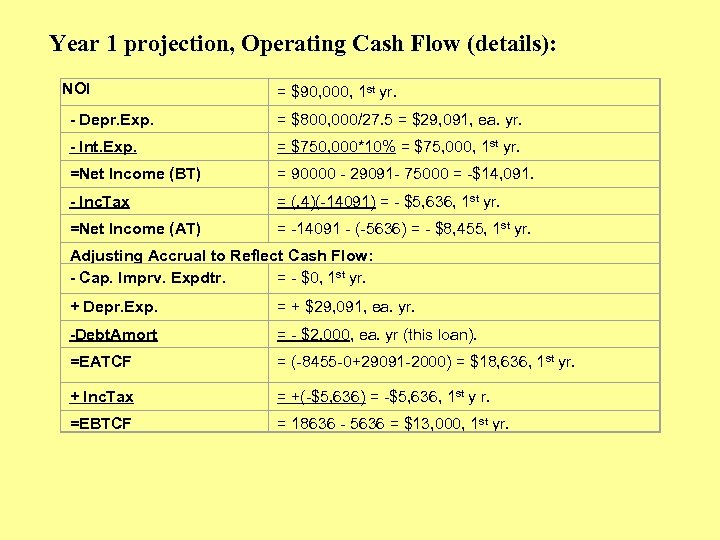Year 1 projection, Operating Cash Flow (details): NOI - Depr. Exp. - Int. Exp. =Net Income (BT) - Inc. Tax =Net Income (AT) = \$90, 000, 1 st yr. = \$800, 000/27. 5 = \$29, 091, ea. yr. = \$750, 000*10% = \$75, 000, 1 st yr. = 90000 - 29091 - 75000 = -\$14, 091. = (. 4)(-14091) = - \$5, 636, 1 st yr. = -14091 - (-5636) = - \$8, 455, 1 st yr. Adjusting Accrual to Reflect Cash Flow: - Cap. Imprv. Expdtr. = - \$0, 1 st yr. + Depr. Exp. -Debt. Amort =EATCF = + \$29, 091, ea. yr. = - \$2, 000, ea. yr (this loan). = (-8455 -0+29091 -2000) = \$18, 636, 1 st yr. + Inc. Tax =EBTCF = +(-\$5, 636) = -\$5, 636, 1 st y r. = 18636 - 5636 = \$13, 000, 1 st yr.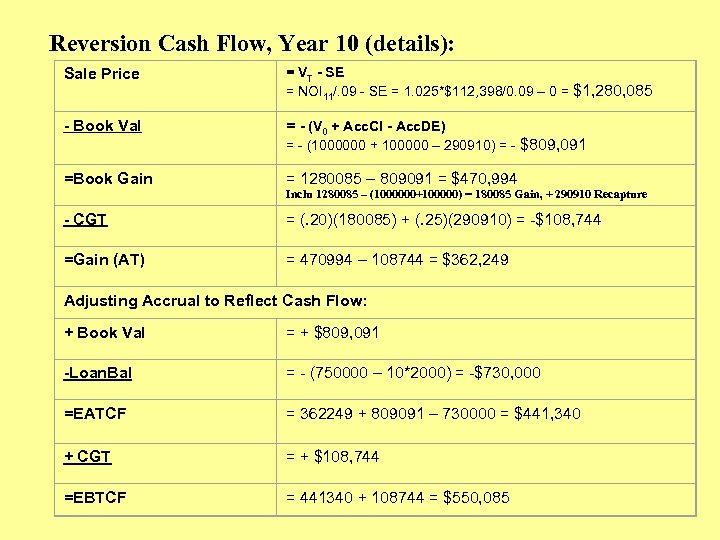Reversion Cash Flow, Year 10 (details): Sale Price = VT - SE = NOI 11/. 09 - SE = 1. 025*\$112, 398/0. 09 – 0 = \$1, 280, 085 - Book Val = - (V 0 + Acc. CI - Acc. DE) = - (1000000 + 100000 – 290910) = - \$809, 091 =Book Gain = 1280085 – 809091 = \$470, 994 Inclu 1280085 – (1000000+100000) = 180085 Gain, + 290910 Recapture - CGT = (. 20)(180085) + (. 25)(290910) = -\$108, 744 =Gain (AT) = 470994 – 108744 = \$362, 249 Adjusting Accrual to Reflect Cash Flow: + Book Val = + \$809, 091 -Loan. Bal = - (750000 – 10*2000) = -\$730, 000 =EATCF = 362249 + 809091 – 730000 = \$441, 340 + CGT = + \$108, 744 =EBTCF = 441340 + 108744 = \$550, 085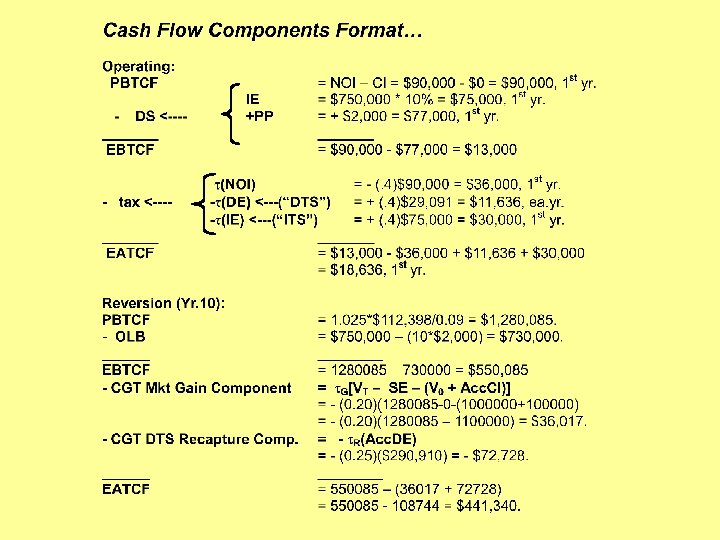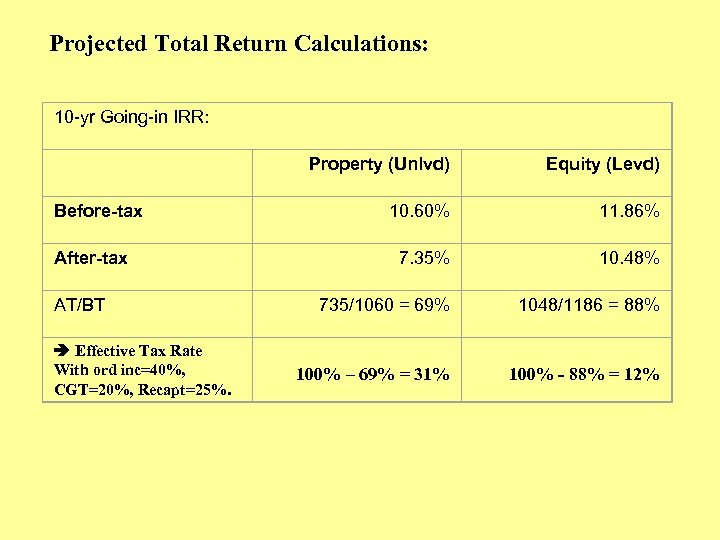Projected Total Return Calculations: 10 -yr Going-in IRR: Before-tax After-tax AT/BT Effective Tax Rate With ord inc=40%, CGT=20%, Recapt=25%. Property (Unlvd) Equity (Levd) 10. 60% 11. 86% 7. 35% 10. 48% 735/1060 = 69% 1048/1186 = 88% 100% – 69% = 31% 100% - 88% = 12%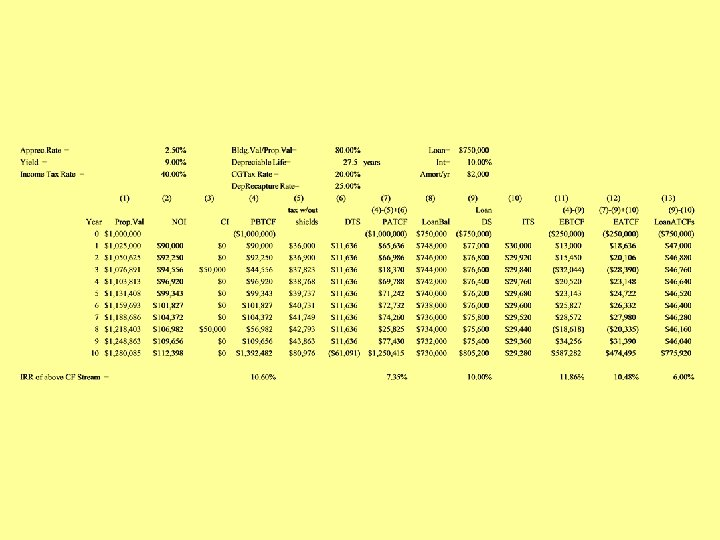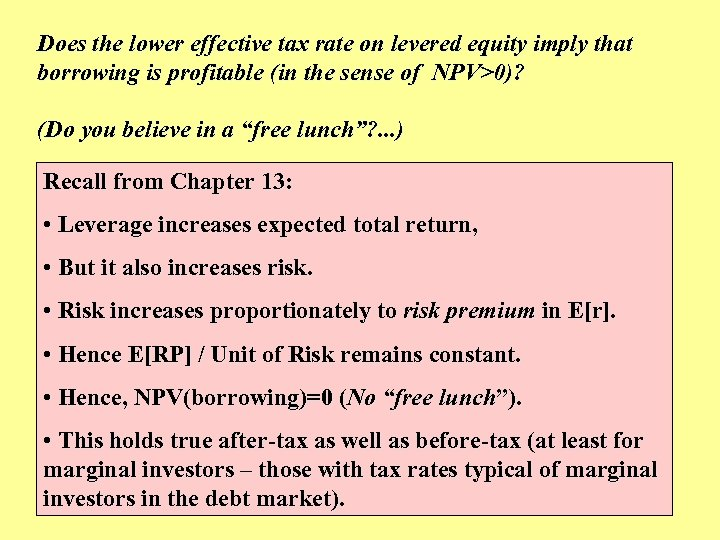Does the lower effective tax rate on levered equity imply that borrowing is profitable (in the sense of NPV>0)? (Do you believe in a “free lunch”? . . . ) Recall from Chapter 13: • Leverage increases expected total return, • But it also increases risk. • Risk increases proportionately to risk premium in E[r]. • Hence E[RP] / Unit of Risk remains constant. • Hence, NPV(borrowing)=0 (No “free lunch”). • This holds true after-tax as well as before-tax (at least for marginal investors – those with tax rates typical of marginal investors in the debt market).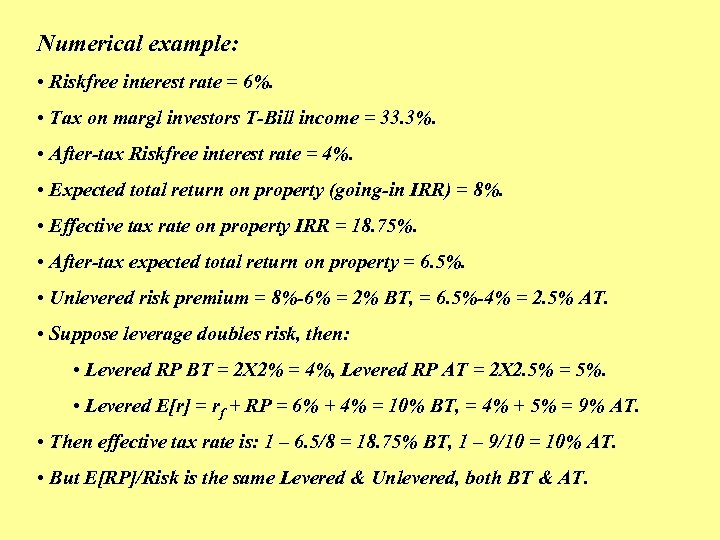Numerical example: • Riskfree interest rate = 6%. • Tax on margl investors T-Bill income = 33. 3%. • After-tax Riskfree interest rate = 4%. • Expected total return on property (going-in IRR) = 8%. • Effective tax rate on property IRR = 18. 75%. • After-tax expected total return on property = 6. 5%. • Unlevered risk premium = 8%-6% = 2% BT, = 6. 5%-4% = 2. 5% AT. • Suppose leverage doubles risk, then: • Levered RP BT = 2 X 2% = 4%, Levered RP AT = 2 X 2. 5% = 5%. • Levered E[r] = rf + RP = 6% + 4% = 10% BT, = 4% + 5% = 9% AT. • Then effective tax rate is: 1 – 6. 5/8 = 18. 75% BT, 1 – 9/10 = 10% AT. • But E[RP]/Risk is the same Levered & Unlevered, both BT & AT.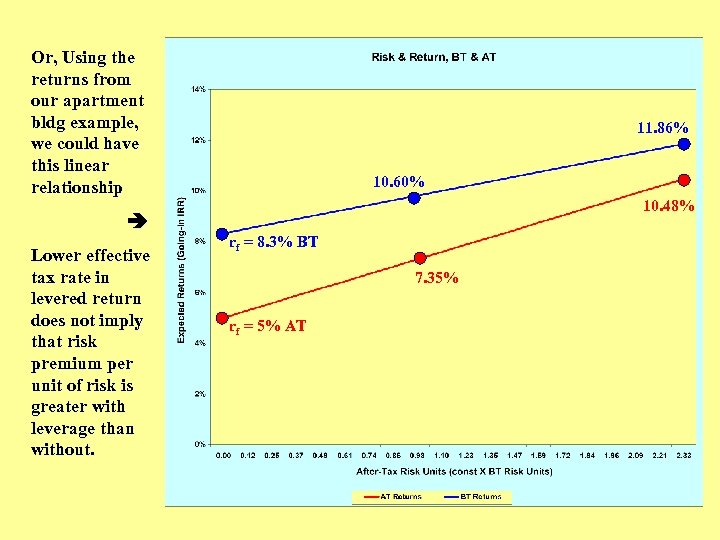Or, Using the returns from our apartment bldg example, we could have this linear relationship Lower effective tax rate in levered return does not imply that risk premium per unit of risk is greater with leverage than without. 11. 86% 10. 60% 10. 48% rf = 8. 3% BT 7. 35% rf = 5% AT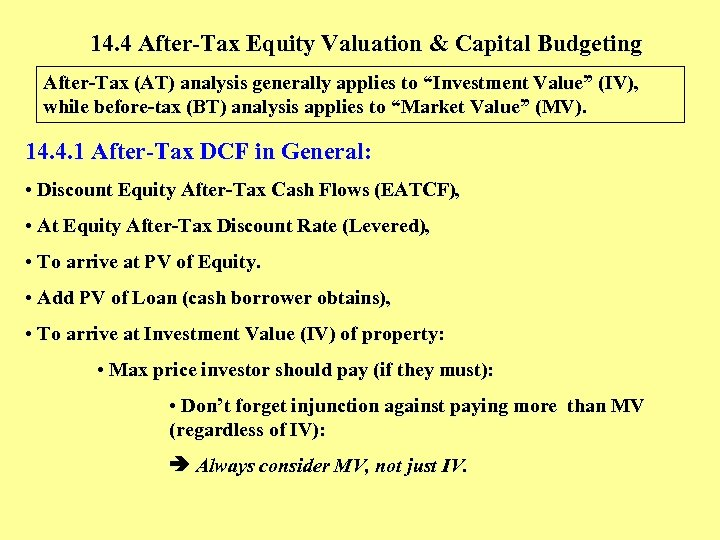14. 4 After-Tax Equity Valuation & Capital Budgeting After-Tax (AT) analysis generally applies to “Investment Value” (IV), while before-tax (BT) analysis applies to “Market Value” (MV). 14. 4. 1 After-Tax DCF in General: • Discount Equity After-Tax Cash Flows (EATCF), • At Equity After-Tax Discount Rate (Levered), • To arrive at PV of Equity. • Add PV of Loan (cash borrower obtains), • To arrive at Investment Value (IV) of property: • Max price investor should pay (if they must): • Don’t forget injunction against paying more than MV (regardless of IV): Always consider MV, not just IV.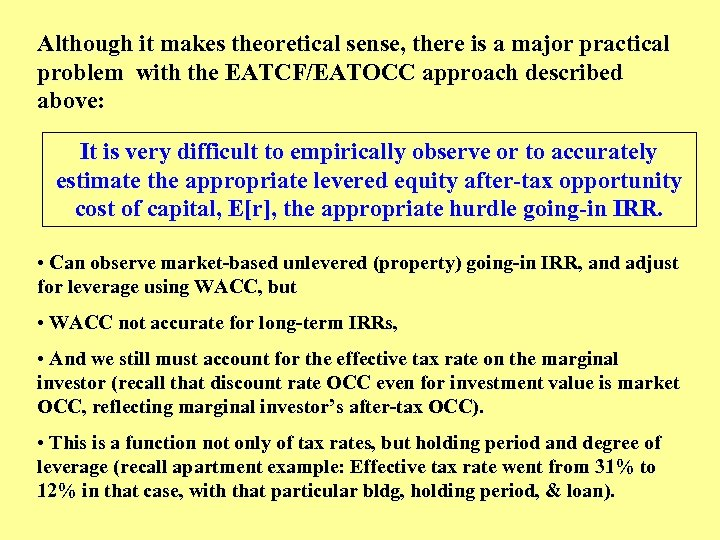Although it makes theoretical sense, there is a major practical problem with the EATCF/EATOCC approach described above: It is very difficult to empirically observe or to accurately estimate the appropriate levered equity after-tax opportunity cost of capital, E[r], the appropriate hurdle going-in IRR. • Can observe market-based unlevered (property) going-in IRR, and adjust for leverage using WACC, but • WACC not accurate for long-term IRRs, • And we still must account for the effective tax rate on the marginal investor (recall that discount rate OCC even for investment value is market OCC, reflecting marginal investor’s after-tax OCC). • This is a function not only of tax rates, but holding period and degree of leverage (recall apartment example: Effective tax rate went from 31% to 12% in that case, with that particular bldg, holding period, & loan).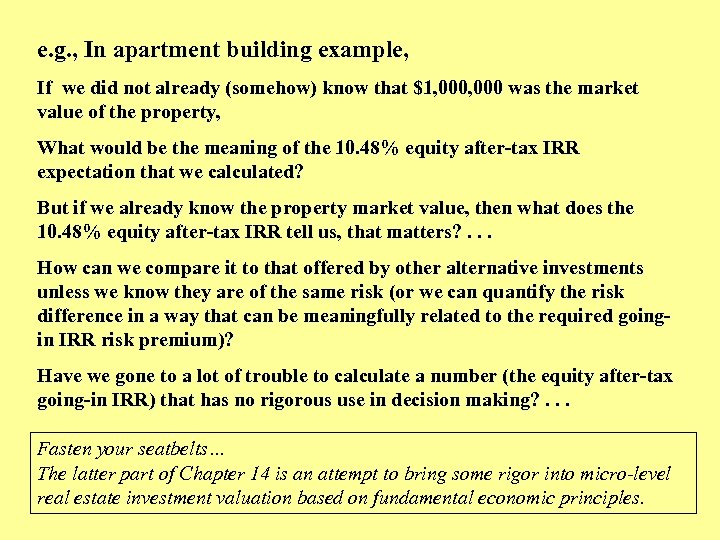e. g. , In apartment building example, If we did not already (somehow) know that \$1, 000 was the market value of the property, What would be the meaning of the 10. 48% equity after-tax IRR expectation that we calculated? But if we already know the property market value, then what does the 10. 48% equity after-tax IRR tell us, that matters? . . . How can we compare it to that offered by other alternative investments unless we know they are of the same risk (or we can quantify the risk difference in a way that can be meaningfully related to the required goingin IRR risk premium)? Have we gone to a lot of trouble to calculate a number (the equity after-tax going-in IRR) that has no rigorous use in decision making? . . . Fasten your seatbelts… The latter part of Chapter 14 is an attempt to bring some rigor into micro-level real estate investment valuation based on fundamental economic principles.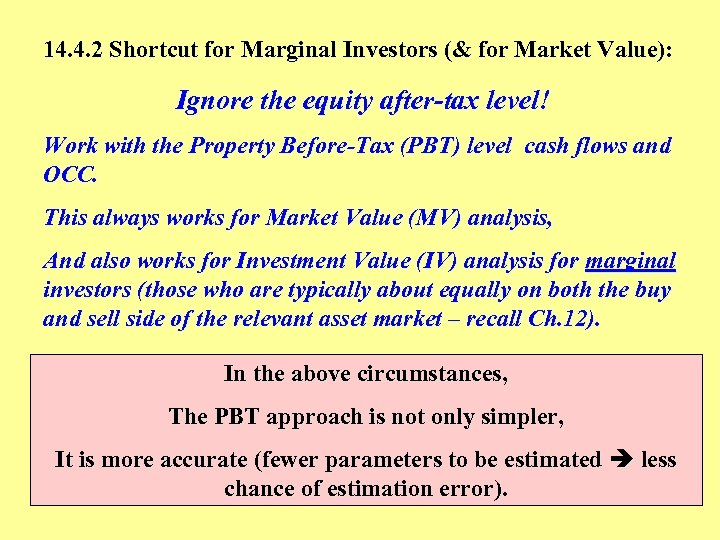14. 4. 2 Shortcut for Marginal Investors (& for Market Value): Ignore the equity after-tax level! Work with the Property Before-Tax (PBT) level cash flows and OCC. This always works for Market Value (MV) analysis, And also works for Investment Value (IV) analysis for marginal investors (those who are typically about equally on both the buy and sell side of the relevant asset market – recall Ch. 12). In the above circumstances, The PBT approach is not only simpler, It is more accurate (fewer parameters to be estimated less chance of estimation error).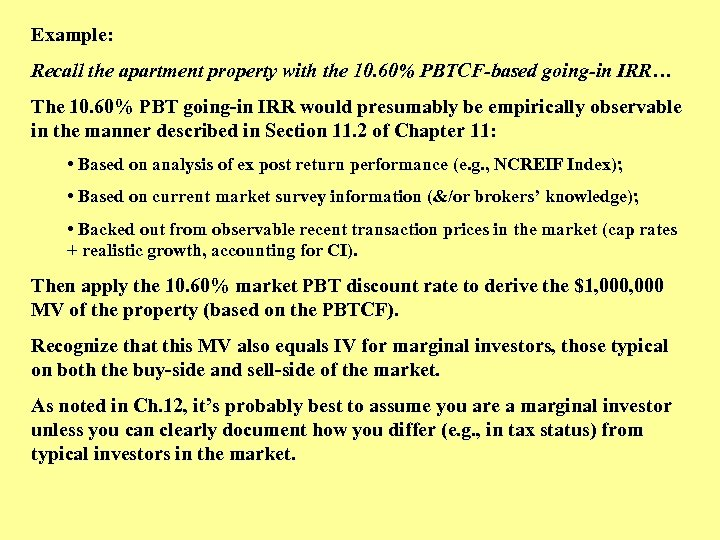Example: Recall the apartment property with the 10. 60% PBTCF-based going-in IRR… The 10. 60% PBT going-in IRR would presumably be empirically observable in the manner described in Section 11. 2 of Chapter 11: • Based on analysis of ex post return performance (e. g. , NCREIF Index); • Based on current market survey information (&/or brokers’ knowledge); • Backed out from observable recent transaction prices in the market (cap rates + realistic growth, accounting for CI). Then apply the 10. 60% market PBT discount rate to derive the \$1, 000 MV of the property (based on the PBTCF). Recognize that this MV also equals IV for marginal investors, those typical on both the buy-side and sell-side of the market. As noted in Ch. 12, it’s probably best to assume you are a marginal investor unless you can clearly document how you differ (e. g. , in tax status) from typical investors in the market.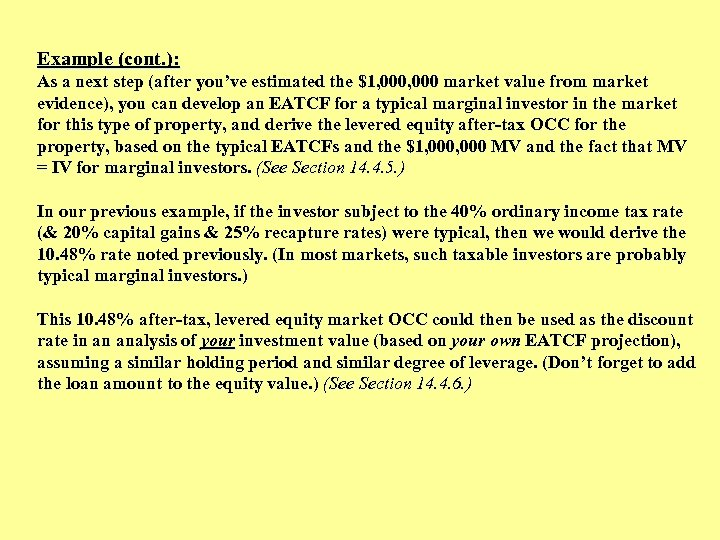Example (cont. ): As a next step (after you’ve estimated the \$1, 000 market value from market evidence), you can develop an EATCF for a typical marginal investor in the market for this type of property, and derive the levered equity after-tax OCC for the property, based on the typical EATCFs and the \$1, 000 MV and the fact that MV = IV for marginal investors. (See Section 14. 4. 5. ) In our previous example, if the investor subject to the 40% ordinary income tax rate (& 20% capital gains & 25% recapture rates) were typical, then we would derive the 10. 48% rate noted previously. (In most markets, such taxable investors are probably typical marginal investors. ) This 10. 48% after-tax, levered equity market OCC could then be used as the discount rate in an analysis of your investment value (based on your own EATCF projection), assuming a similar holding period and similar degree of leverage. (Don’t forget to add the loan amount to the equity value. ) (See Section 14. 4. 6. )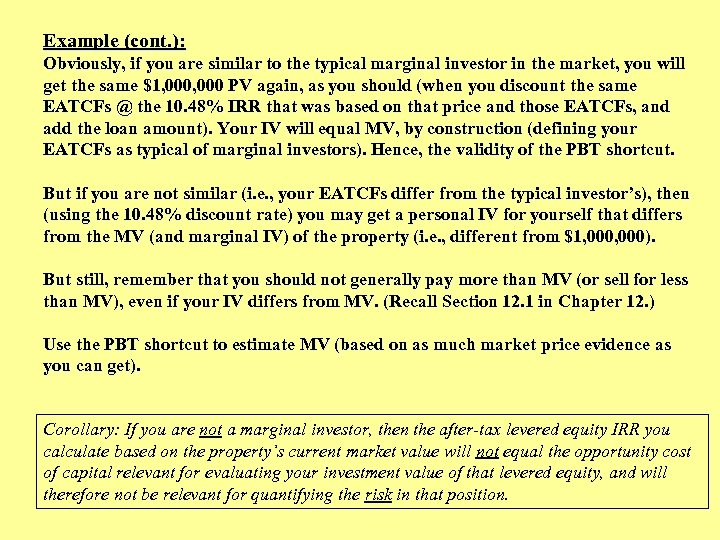Example (cont. ): Obviously, if you are similar to the typical marginal investor in the market, you will get the same \$1, 000 PV again, as you should (when you discount the same EATCFs @ the 10. 48% IRR that was based on that price and those EATCFs, and add the loan amount). Your IV will equal MV, by construction (defining your EATCFs as typical of marginal investors). Hence, the validity of the PBT shortcut. But if you are not similar (i. e. , your EATCFs differ from the typical investor’s), then (using the 10. 48% discount rate) you may get a personal IV for yourself that differs from the MV (and marginal IV) of the property (i. e. , different from \$1, 000). But still, remember that you should not generally pay more than MV (or sell for less than MV), even if your IV differs from MV. (Recall Section 12. 1 in Chapter 12. ) Use the PBT shortcut to estimate MV (based on as much market price evidence as you can get). Corollary: If you are not a marginal investor, then the after-tax levered equity IRR you calculate based on the property’s current market value will not equal the opportunity cost of capital relevant for evaluating your investment value of that levered equity, and will therefore not be relevant for quantifying the risk in that position.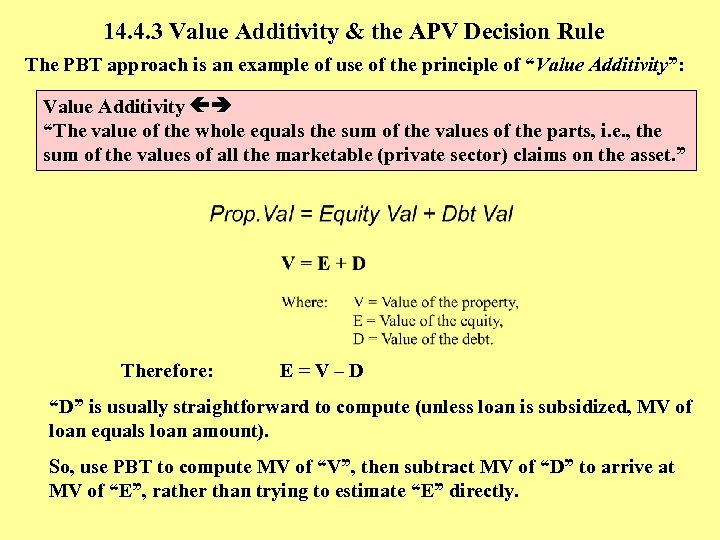14. 4. 3 Value Additivity & the APV Decision Rule The PBT approach is an example of use of the principle of “Value Additivity”: Value Additivity “The value of the whole equals the sum of the values of the parts, i. e. , the sum of the values of all the marketable (private sector) claims on the asset. ” Therefore: E=V–D “D” is usually straightforward to compute (unless loan is subsidized, MV of loan equals loan amount). So, use PBT to compute MV of “V”, then subtract MV of “D” to arrive at MV of “E”, rather than trying to estimate “E” directly.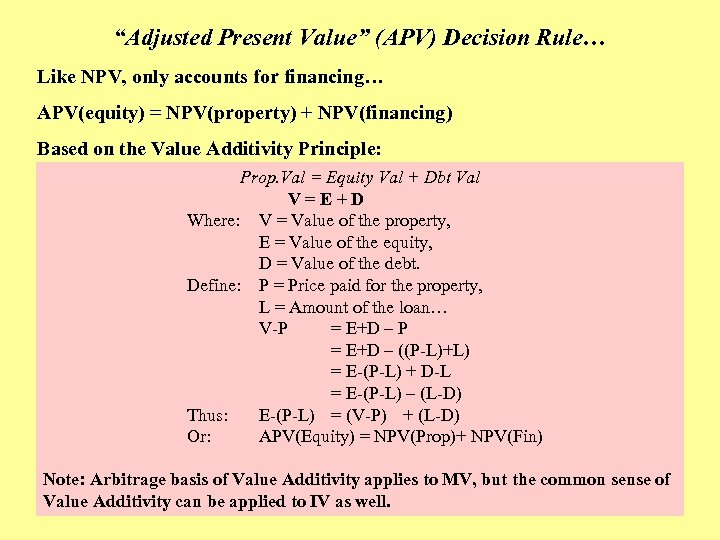“Adjusted Present Value” (APV) Decision Rule… Like NPV, only accounts for financing… APV(equity) = NPV(property) + NPV(financing) Based on the Value Additivity Principle: Prop. Val = Equity Val + Dbt Val V=E+D Where: V = Value of the property, E = Value of the equity, D = Value of the debt. Define: P = Price paid for the property, L = Amount of the loan… V-P = E+D – ((P-L)+L) = E-(P-L) + D-L = E-(P-L) – (L-D) Thus: E-(P-L) = (V-P) + (L-D) Or: APV(Equity) = NPV(Prop)+ NPV(Fin) Note: Arbitrage basis of Value Additivity applies to MV, but the common sense of Value Additivity can be applied to IV as well.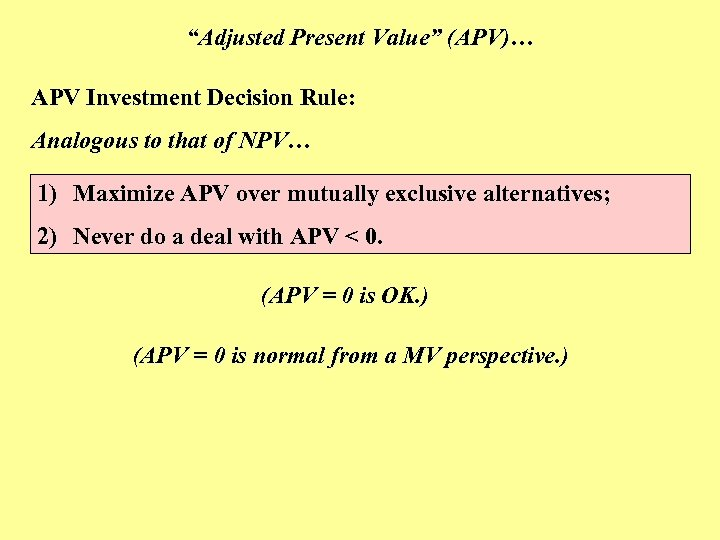“Adjusted Present Value” (APV)… APV Investment Decision Rule: Analogous to that of NPV… 1) Maximize APV over mutually exclusive alternatives; 2) Never do a deal with APV < 0. (APV = 0 is OK. ) (APV = 0 is normal from a MV perspective. )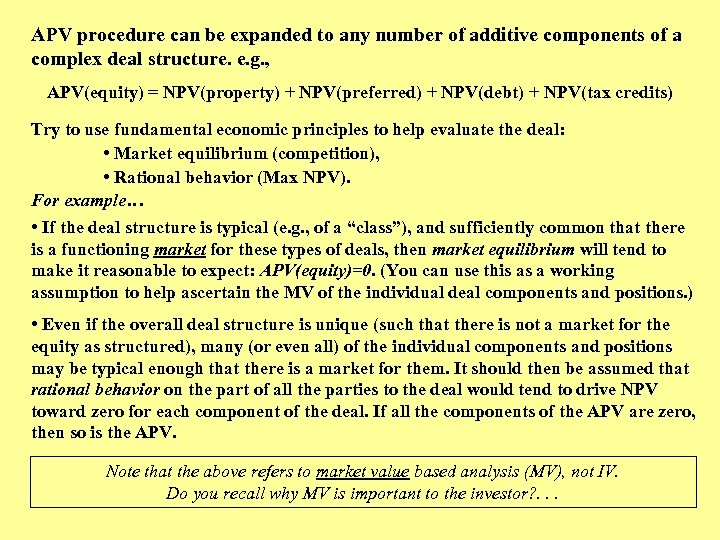APV procedure can be expanded to any number of additive components of a complex deal structure. e. g. , APV(equity) = NPV(property) + NPV(preferred) + NPV(debt) + NPV(tax credits) Try to use fundamental economic principles to help evaluate the deal: • Market equilibrium (competition), • Rational behavior (Max NPV). For example… • If the deal structure is typical (e. g. , of a “class”), and sufficiently common that there is a functioning market for these types of deals, then market equilibrium will tend to make it reasonable to expect: APV(equity)=0. (You can use this as a working assumption to help ascertain the MV of the individual deal components and positions. ) • Even if the overall deal structure is unique (such that there is not a market for the equity as structured), many (or even all) of the individual components and positions may be typical enough that there is a market for them. It should then be assumed that rational behavior on the part of all the parties to the deal would tend to drive NPV toward zero for each component of the deal. If all the components of the APV are zero, then so is the APV. Note that the above refers to market value based analysis (MV), not IV. Do you recall why MV is important to the investor? . . .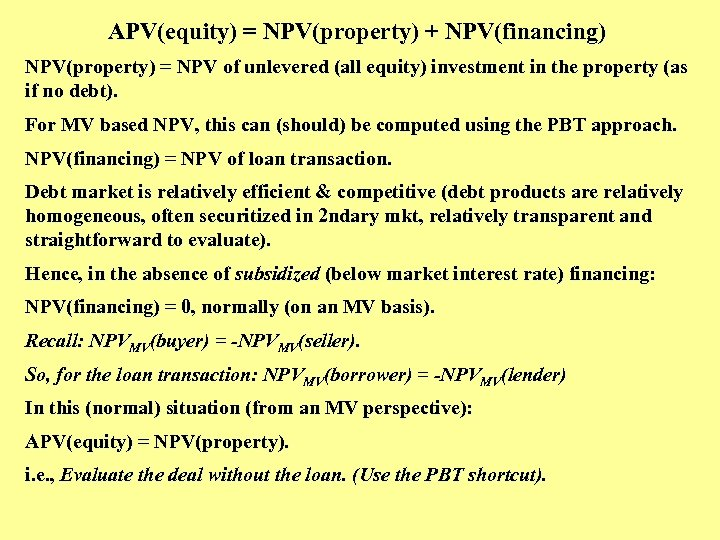APV(equity) = NPV(property) + NPV(financing) NPV(property) = NPV of unlevered (all equity) investment in the property (as if no debt). For MV based NPV, this can (should) be computed using the PBT approach. NPV(financing) = NPV of loan transaction. Debt market is relatively efficient & competitive (debt products are relatively homogeneous, often securitized in 2 ndary mkt, relatively transparent and straightforward to evaluate). Hence, in the absence of subsidized (below market interest rate) financing: NPV(financing) = 0, normally (on an MV basis). Recall: NPVMV(buyer) = -NPVMV(seller). So, for the loan transaction: NPVMV(borrower) = -NPVMV(lender) In this (normal) situation (from an MV perspective): APV(equity) = NPV(property). i. e. , Evaluate the deal without the loan. (Use the PBT shortcut).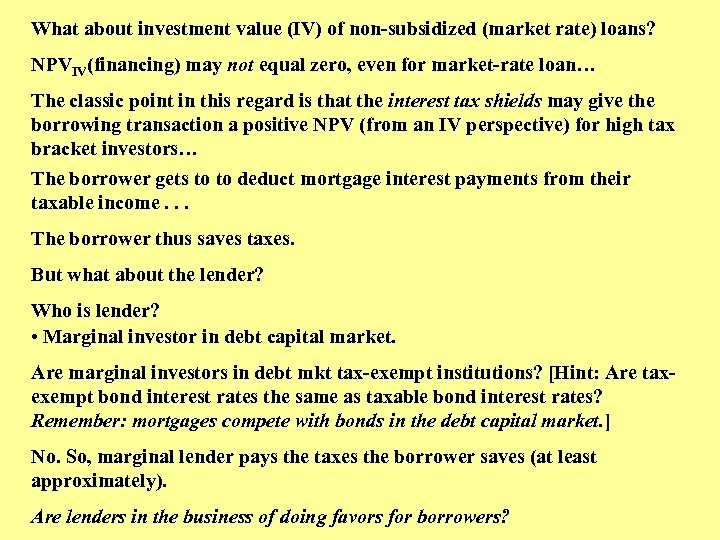What about investment value (IV) of non-subsidized (market rate) loans? NPVIV(financing) may not equal zero, even for market-rate loan… The classic point in this regard is that the interest tax shields may give the borrowing transaction a positive NPV (from an IV perspective) for high tax bracket investors… The borrower gets to to deduct mortgage interest payments from their taxable income. . . The borrower thus saves taxes. But what about the lender? Who is lender? • Marginal investor in debt capital market. Are marginal investors in debt mkt tax-exempt institutions? [Hint: Are taxexempt bond interest rates the same as taxable bond interest rates? Remember: mortgages compete with bonds in the debt capital market. ] No. So, marginal lender pays the taxes the borrower saves (at least approximately). Are lenders in the business of doing favors for borrowers?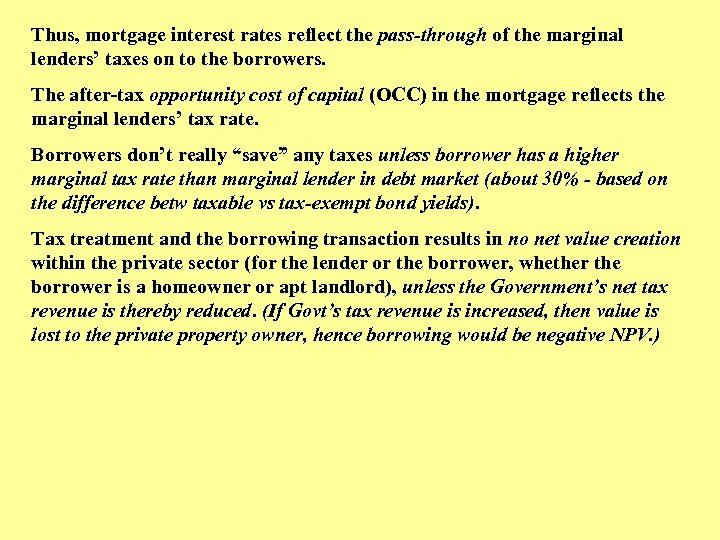Thus, mortgage interest rates reflect the pass-through of the marginal lenders’ taxes on to the borrowers. The after-tax opportunity cost of capital (OCC) in the mortgage reflects the marginal lenders’ tax rate. Borrowers don’t really “save” any taxes unless borrower has a higher marginal tax rate than marginal lender in debt market (about 30% - based on the difference betw taxable vs tax-exempt bond yields). Tax treatment and the borrowing transaction results in no net value creation within the private sector (for the lender or the borrower, whether the borrower is a homeowner or apt landlord), unless the Government’s net tax revenue is thereby reduced. (If Govt’s tax revenue is increased, then value is lost to the private property owner, hence borrowing would be negative NPV. )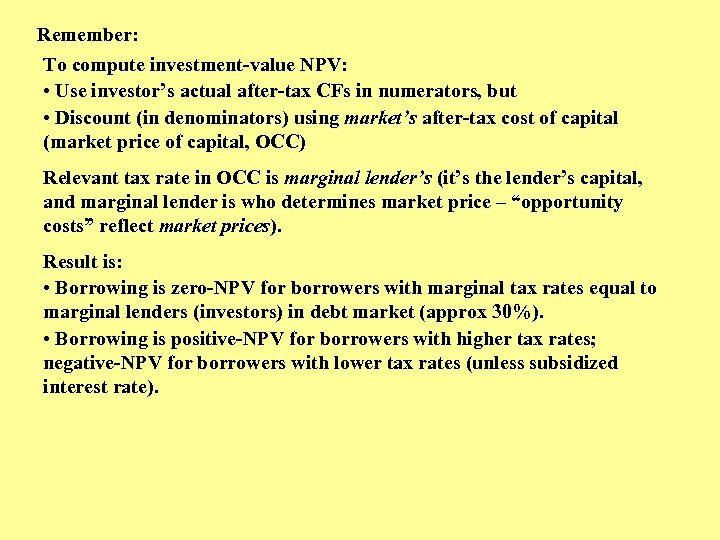Remember: To compute investment-value NPV: • Use investor’s actual after-tax CFs in numerators, but • Discount (in denominators) using market’s after-tax cost of capital (market price of capital, OCC) Relevant tax rate in OCC is marginal lender’s (it’s the lender’s capital, and marginal lender is who determines market price – “opportunity costs” reflect market prices). Result is: • Borrowing is zero-NPV for borrowers with marginal tax rates equal to marginal lenders (investors) in debt market (approx 30%). • Borrowing is positive-NPV for borrowers with higher tax rates; negative-NPV for borrowers with lower tax rates (unless subsidized interest rate).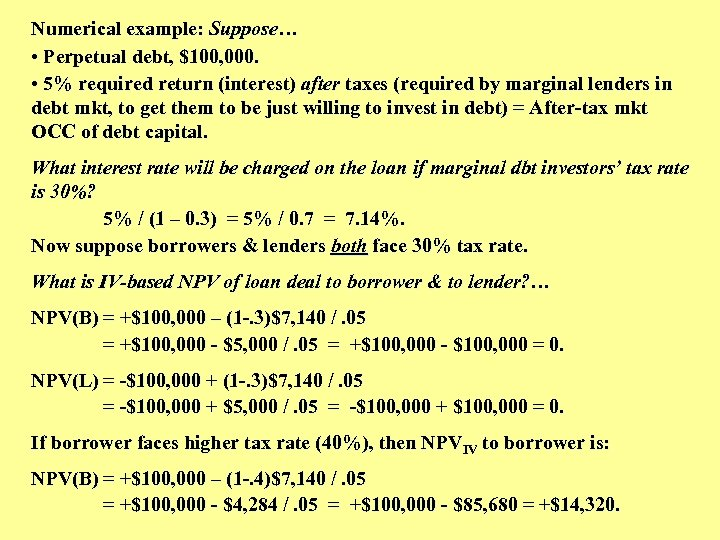Numerical example: Suppose… • Perpetual debt, \$100, 000. • 5% required return (interest) after taxes (required by marginal lenders in debt mkt, to get them to be just willing to invest in debt) = After-tax mkt OCC of debt capital. What interest rate will be charged on the loan if marginal dbt investors’ tax rate is 30%? 5% / (1 – 0. 3) = 5% / 0. 7 = 7. 14%. Now suppose borrowers & lenders both face 30% tax rate. What is IV-based NPV of loan deal to borrower & to lender? … NPV(B) = +\$100, 000 – (1 -. 3)\$7, 140 /. 05 = +\$100, 000 - \$5, 000 /. 05 = +\$100, 000 - \$100, 000 = 0. NPV(L) = -\$100, 000 + (1 -. 3)\$7, 140 /. 05 = -\$100, 000 + \$5, 000 /. 05 = -\$100, 000 + \$100, 000 = 0. If borrower faces higher tax rate (40%), then NPVIV to borrower is: NPV(B) = +\$100, 000 – (1 -. 4)\$7, 140 /. 05 = +\$100, 000 - \$4, 284 /. 05 = +\$100, 000 - \$85, 680 = +\$14, 320.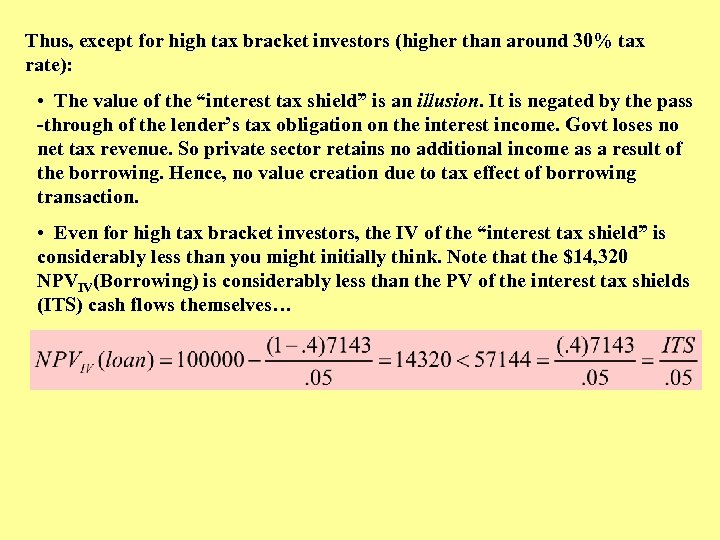Thus, except for high tax bracket investors (higher than around 30% tax rate): • The value of the “interest tax shield” is an illusion. It is negated by the pass -through of the lender’s tax obligation on the interest income. Govt loses no net tax revenue. So private sector retains no additional income as a result of the borrowing. Hence, no value creation due to tax effect of borrowing transaction. • Even for high tax bracket investors, the IV of the “interest tax shield” is considerably less than you might initially think. Note that the \$14, 320 NPVIV(Borrowing) is considerably less than the PV of the interest tax shields (ITS) cash flows themselves…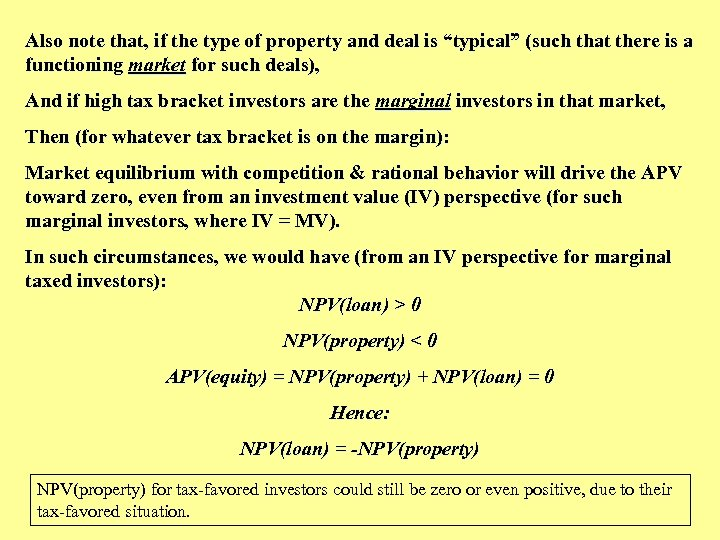Also note that, if the type of property and deal is “typical” (such that there is a functioning market for such deals), And if high tax bracket investors are the marginal investors in that market, Then (for whatever tax bracket is on the margin): Market equilibrium with competition & rational behavior will drive the APV toward zero, even from an investment value (IV) perspective (for such marginal investors, where IV = MV). In such circumstances, we would have (from an IV perspective for marginal taxed investors): NPV(loan) > 0 NPV(property) < 0 APV(equity) = NPV(property) + NPV(loan) = 0 Hence: NPV(loan) = -NPV(property) for tax-favored investors could still be zero or even positive, due to their tax-favored situation.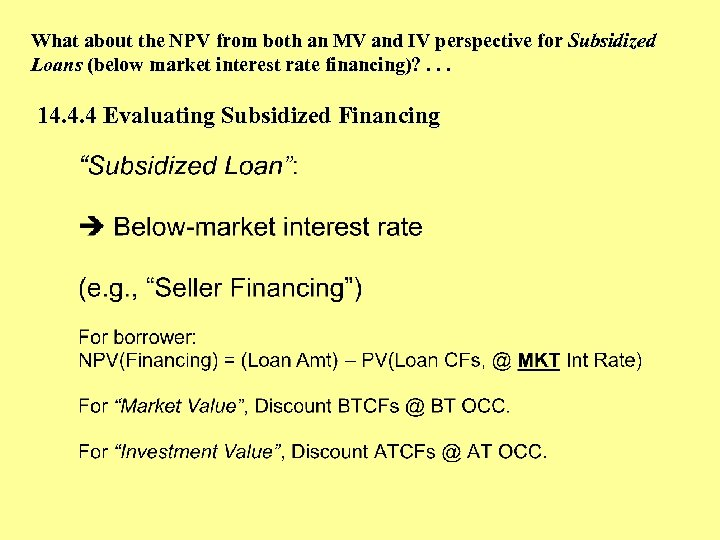What about the NPV from both an MV and IV perspective for Subsidized Loans (below market interest rate financing)? . . . 14. 4. 4 Evaluating Subsidized Financing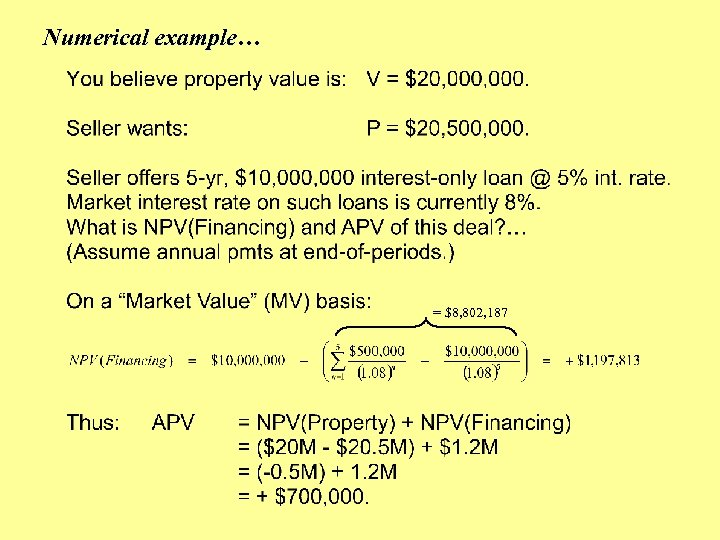Numerical example… = \$8, 802, 187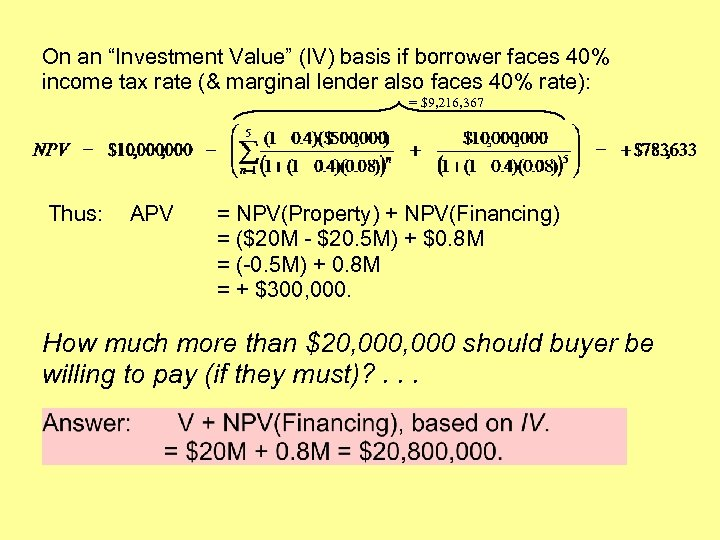On an “Investment Value” (IV) basis if borrower faces 40% income tax rate (& marginal lender also faces 40% rate): = \$9, 216, 367 Thus: APV = NPV(Property) + NPV(Financing) = (\$20 M - \$20. 5 M) + \$0. 8 M = (-0. 5 M) + 0. 8 M = + \$300, 000. How much more than \$20, 000 should buyer be willing to pay (if they must)? . . .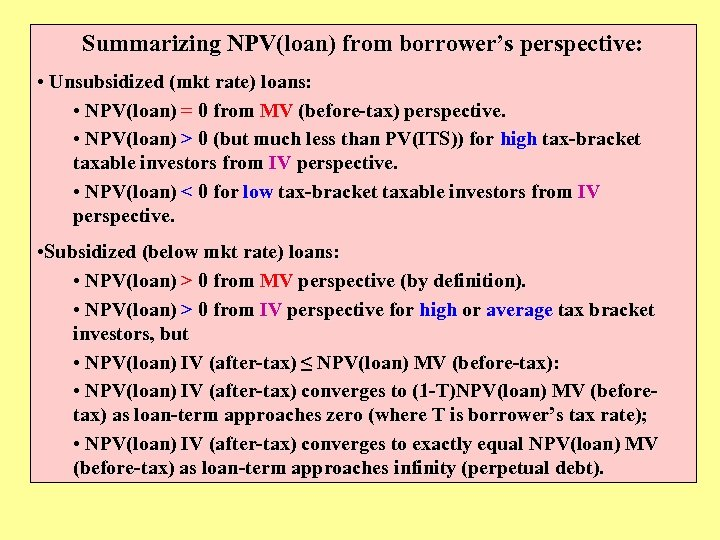Summarizing NPV(loan) from borrower’s perspective: • Unsubsidized (mkt rate) loans: • NPV(loan) = 0 from MV (before-tax) perspective. • NPV(loan) > 0 (but much less than PV(ITS)) for high tax-bracket taxable investors from IV perspective. • NPV(loan) < 0 for low tax-bracket taxable investors from IV perspective. • Subsidized (below mkt rate) loans: • NPV(loan) > 0 from MV perspective (by definition). • NPV(loan) > 0 from IV perspective for high or average tax bracket investors, but • NPV(loan) IV (after-tax) ≤ NPV(loan) MV (before-tax): • NPV(loan) IV (after-tax) converges to (1 -T)NPV(loan) MV (beforetax) as loan-term approaches zero (where T is borrower’s tax rate); • NPV(loan) IV (after-tax) converges to exactly equal NPV(loan) MV (before-tax) as loan-term approaches infinity (perpetual debt).